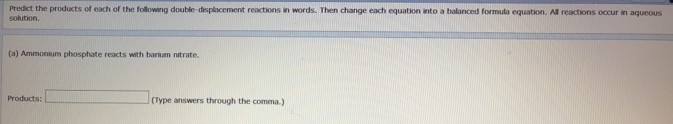# Problem: Predict the products of each of the following double-displacement reactions in words. Then change each equation into a balanced formula equation. All reactions occur in aqueous solution. Ammonium phosphate reacts with barium nitrate.

###### FREE Expert Solution###### Problem Details

Predict the products of each of the following double-displacement reactions in words. Then change each equation into a balanced formula equation. All reactions occur in aqueous solution.

Ammonium phosphate reacts with barium nitrate.